GeoGebra in project-based learning (Geo-PjBL): A dynamic tool for analytical geometry course

GEOGEBRA IN PROJECT-BASED LEARNING (GEO-PJBL):
A DYNAMIC TOOL FOR ANALYTICAL GEOMETRY COURSE

1Mathematics Education - Universitas Muhammadiyah Surakarta (Indonesia)
2Primary Education - Universitas PGRI Madiun (Indonesia)
3Mathematics Education - Universitas PGRI Madiun (Indonesia)

Accepted September 2021

Abstract

The integration of learning models and software is a trend in mathematics courses. However, no existing learning model for geometry courses involves the students in the making of a tool or media project. The researchers noticed the potential of the project-based learning (PjBL) model and GeoGebra in analytical geometry courses. This study revealed differences in the influence of the Geo-PjBL and PjBL models on students’ achievement. The subjects consisted of 137 prospective mathematics teachers. The Basic Geometry Instrument (BGI) was used to measure the subjects’ initial ability in basic geometry, and the Geometry Analytic Instrument (GAI) was used to evaluate the model and prospective teachers’ performance. The Geo-PjBL and PjBL classroom activities lasted for 15 weeks. Both classrooms received the same content; the difference between the Geo-PjBL and PjBL classrooms was the tools used to present the problems and the project results. An analysis of covariance (ANCOVA) was conducted to analyze the data (α = 0.01). The Geo-PjBL model is more effective in applying analytical geometry subjects that require precision and accurate visual illustrations. Meanwhile, in the range of algebraic operations, the Geo-PjBL model is as effective as the PjBL model.

Keywords – GeoGebra, Project-based learning, Dynamic tool, Analytical geometry.

 Kholid, M.N., Pradana, L.N., Maharani, S., & Swastika, A. (2022). GeoGebra in project-based learning (Geo-PjBL): A dynamic tool for analytical geometry course. Journal of Technology and Science Education, 12(1), 112-120. https://doi.org/10.3926/jotse.1267

----------

1. 1. Introduction

Geometry is the science of mathematics in the spatial domain (Casa, Firmender, Gavin & Carroll, 2016). A prospective mathematics teacher in Indonesia is required to learn about and teach geometry. The researchers conducted investigations regarding geometry courses at this level and determined the average scores of some geometry courses in the last five years. The studies included plane, space, analytical, and non-Euclidean geometry. Figure 1 shows that over the last five years, the average score in analytical geometry courses is lower than those in other geometry courses. Although the students’ basic geometry abilities are relatively good, the students face difficulties in analytical geometry courses.

The researchers also reviewed various prior studies and categorized them into two focuses. First, studies focusing on the development or application of learning models on geometry include the following: deep learning 3D-shaped surfaces (Sinha, Bai & Ramani, 2016), model-based deep hand pose estimation (Zhou, Wan, Wei, Xue & Wei, 2016), the flipped learning model (Voronina, Moroz, Sudarikov, Rakhimzhanova & Muratbakeev, 2017), and discovery learning using a scientific approach (Ummah, Inam & Azmi, 2019). Second, studies that develop or apply tools in geometry learning include GCLC (Janičić, 2006), an augmented reality–assisted learning system (Lin, Chen & Chang, 2015), context-aware ubiquitous learning (Crompton, 2015), BLOG-GOTHIC (Indahwati & Tafrilyanto, 2018), Wingeom Software (Fonna & Mursalin, 2019), and Scrap-Mod (Hidayah & Destari, 2019).

Based on these studies, no existing learning model for geometry courses involves the students in the making of a tool or media as a learning project. The researchers noticed the potential of the project-based learning (PjBL) model and GeoGebra in analytical geometry courses. By integrating the PjBL model and GeoGebra (Geo-PjBL), the study aims to reveal whether there are differences in the influence of the Geo-PjBL and PjBL models on the learning achievement of analytic geometry courses for prospective mathematics teachers. The quantitative data was observed according to actual conditions.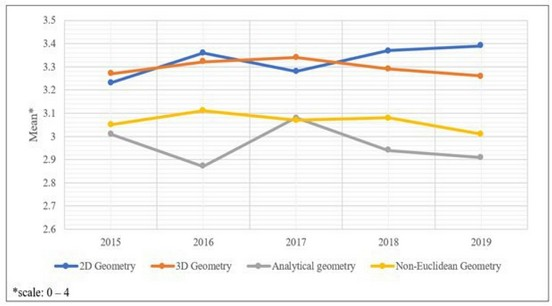Figure 1. Average geometry scores (2015–2019)

2. Methodology

2.1. Research Goal and Design

This study utilized quasi-experimental research to examine the effectiveness of the Geo-PjBL model in an analytical geometry course. The first phase of the study was collecting data on the initial performances and achievements of the students employed to determine consistency in the data before the beginning of the course. The second phase lasted for 15 weeks (Geo-PjBL classroom and PjBL classroom). Every five weeks, the researchers evaluated the students by following their progress in the analytical geometry course. In the third phase, the researchers then analyzed the data from the students’ test results to examine the effectiveness of the Geo-PjBL model in the course.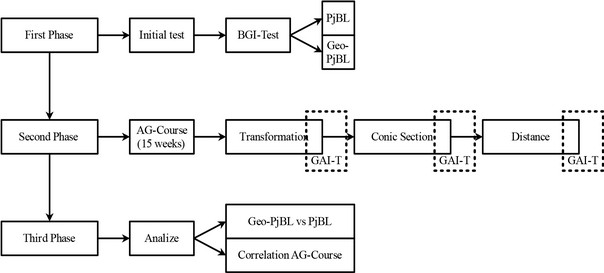Figure 2. Research design model

2.2. Participants

The participants in this study were prospective teachers in the Department of Mathematics Education, Central Java, Indonesia. A total of 137 prospective teachers were enrolled in six classrooms. Each classroom was labeled either Geo-PjBL or PjBL. The Geo-PjBL classroom included 63 prospective teachers (mean age = 19.32; range age = 18.74-20.21), and the PjBL classroom included 74 prospective teachers (mean age = 19.36; range age = 18.66-20.12). The Geo-PjBL classroom used project-based learning with GeoGebra as a tool to present the problem and the project. Meanwhile, the PjBL classroom used project-based learning with paper-based illustrations to present the problem and the project. The researchers controlled the classroom conditions statistically via mean class sizes and mean age, and no significant difference existed between mean class sizes (F (1, 6) = 4.79, p = 0.38) and mean age (F 1, 136) = 4.82, p = 0.13). Apart from including prospective teachers as the participants, this study involved two lecturers in the Geo-PjBL and PjBL classrooms with at least eight years of experience in teaching analytical geometry.

2.3. Design Activity

The analytic geometry course activities used the PjBL model and GeoGebra (Geo-PjBL) as tools. The projects in the learning activities aimed to solve problems related to analytic geometry by presenting various perspectives in the GeoGebra software. GeoGebra has an advantage in delivering spatial objects dynamically to display objects (points, lines, and fields in space) from multiple perspectives.

2.4. Material

Two instruments were administered in this study: the Basic Geometry Instrument (BGI) and the Geometry Analytic Instrument (GAI). The BGI was conducted to measure the college students’ initial ability in basic geometry (2D and 3D geometry). The 30-item multiple-choice test, producing a maximum score of 20, was administered in 60 minutes and conducted two weeks before the design activity began. The internal reliability of this test was 0.711. The GAI, used to evaluate the model and prospective teachers’ performance, included three subjects with different item characteristics. Each scope has a seven‑item essay test; the GAI has a 21-item essay test. The internal reliability of each subject is shown in Table 1. The items in the GAI are non-dichotomously scored (the maximum score of each range is 140). The GAI was administered at different times for each subject. The participants were tested in each classroom, and the test session lasted approximately 70 minutes.

 Subject Item Characteristics Transformation (r = 0.821) Identifying and constructing reflection, rotation, translation, and enlargement Conic Section (r = 0.736) Drawing and analyzing the circle, ellipse, parabola, and hyperbola Distance (r = 0.758) Defining and measuring the distances of points, lines, and shapes

Table 1. Subjects in the Geometry Analytic Instrument

2.5. Procedure

At the beginning of the study, the prospective teachers carried out the BGI as an initial test. Two weeks after the test, the Geo-PjBL and PjBL classroom activities began. Completing all the activities and the three GAI tests took 15 weeks. Figure 3 illustrates that each transformation, conic section, and distance activity was conducted in four weeks. Both classrooms received the same content; the difference between the Geo-PjBL and PjBL classrooms was the tools to present the problems and the project results. The weekly activity lasted 100 minutes, and every five weeks, the GAI was administered to evaluate each subject. In the fifth week, [GAI] Transformation was distributed to the prospective teachers, with [GAI] Conic Section administered in the tenth week and [GAI] Distance in the fifteenth week.

2.6. Data Analysis

Data analysis was used to show the effectiveness of the Geo-PjBL and PjBL activities in the classroom in terms of three subjects. An analysis of covariance (ANCOVA) was conducted to analyze the data (α = 0.01) and for two main purposes. First, the ANCOVA was used to compare the means of the prospective teachers’ performance in each subject. Data on each range was collected via the GAI test results. Second, the ANCOVA was used to find the correlation among the teachers’ performances in the three tests. Apart from the top values, the effect sizes are reported.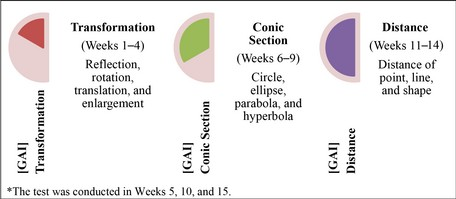Figure 3. Schedule of model and non-model classroom

3. Findings and Discussion

The results are presented in two main sections. They compare the means of the two classes and the correlation among the three subjects.

3.1. The Consistency Model Activity

After the BGI was conducted two weeks before activities began, the researchers compared the means of the two classes (Geo-PjBL and PjBL classrooms). No significant difference was found between the prospective teachers’ performances before each activity (F (1, 136) = 2.63, p = 0.200). Thus, both groups of prospective teachers in the Geo-PjBL and PjBL classrooms displayed the same performance in the BGI.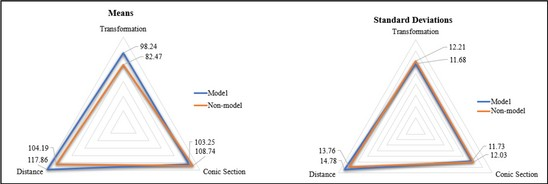Figure 4. Means and standard deviations of the GAI test

 Class df F p Decision Geo-PjBL vs PjBL (1, 136) 2.63 0.200 H0 accepted

Table 2. Results of Consistency Test

3.2. The Effectiveness Model Activity

These results report the effectiveness of the Geo-PjBL and PjBL classroom activities by comparing the means of the GAI test results. With respect to [GAI] Transformation, a significant difference was found in the mean scores between the Geo-PjBL and PjBL classrooms (F (1, 136) = 13.84, p < 0.01); the performance of the prospective teachers in the Geo-PjBL activities was better than that in the PjBL activities. In [GAI] Conic Section, no significant difference was found between the mean test scores (F (1, 136) = 4.97, p = 0.042). Thus, both groups of prospective teachers in the two classes showed the same performance in the conic section activities. Furthermore, in [GAI] Distance, a significant difference was found in the mean test scores (F (1, 136) = 9.58, p < 0.01); the performance of the prospective teachers in the Geo-PjBL activities was better than that in the PjBL activities. The results were unique because two categories favored prospective teachers in the Geo-PjBL classroom (transformation and distance) and there was one category wherein both groups of teachers had the same performance (conic section).

 Geo-PjBL vs PjBL df F p Decision [GAI] Transformation (1, 136) 13.84 <0.01 H0 rejected [GAI] Conic Section (1, 136) 4.97 0.042 H0 accepted [GAI] Distance (1, 136) 9.58 <0.01 H0 rejected

Table 3. Effectiveness of Geo-PjBL

3.3. The Correlation among the Test Subjects

In terms of Transformation × Conic Section, no correlation was found between performances in the subjects (t (135) = 1.67, r = 0.211, p = 0.173). In terms of Transformation × Distance, a positive correlation was found between performances in the subjects (t (135) = 4.18, r = 0.635, p < 0.01). Thus, if the prospective teachers displayed good performance in the transformation subject, they did the same in the distance subject. Furthermore, in terms of Conic Section × Distance, no correlation was found between performances in the subjects (t (135) = 1.48, r = 0.014, p = 0.193).

 Correlation df t r (Pearson) p Decision Transformation × Conic Section 135 1.67 0.211 0.173 H0 accepted Transformation × Distance 135 4.18 0.635 <0.01 H0 rejected Conic Section × Distance 135 1.48 0.014 0.193 H0 accepted

Table 4. Effectiveness of Geo-PjBL

3.4. Discussions

The results showed that the prospective teachers’ achievements in the transformation and distance subjects were better on the Geo-PJBL model. In acquiring transformations and distances, they need accurate visualizations so that the results of the transformation and distance calculations can be realized. GeoGebra in the Geo-PJBL class is invaluable to them in solving transformation and distance problems; thus, their achievements in the Geo-PJBL class are more reliable. As a result, the Geo-PjBL model greatly aids prospective teachers in making graphical illustrations of the problems. It is relevant to note in the research that visual graphics help learners achieve more understanding of the subject (Janičić, 2006; Misrom, Abdurrahman, Abdullah, Osman, Hamzah & Fauzan, 2020; Pradana, Sholikhah, Maharani & Kholid, 2020). Meanwhile, the prospective teachers’ performance in the conic section subject showed no difference because the prospective mathematics teacher uses more algebra operations to identify each conic section’s characteristics. Precise and accurate visual illustrations are not necessary; by creating a sketch (Kholid, Sa’dijah, Hidayanto & Permadi, 2020), the teacher can solve the problem. Therefore, the Geo-PjBL model is more effective in subjects that require a lot of visual illustrations. Students can create such illustrations using software, in this case GeoGebra. This is relevant to the research of Fatahillah, Puspitasari and Hussen (2020), in which GeoGebra is helpful to improve both students' performances and conceptual understanding (Kholid, Imawati, Swastika, Maharani & Pradana, 2021). It is because GeoGebra provides perfect visualization in geometry (Sholihah & Maryono, 2020).

 Problem: What is the particular position of V: x + y + z = 0? Project in Geo-PjBL Class: Student’s Answer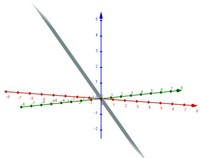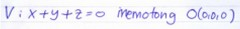Translated Version: V intersects the starting point O(0,0,0). (a)Student’s worksheet in Geo-PjBL class with dynamic tool. Problem: What is the particular position of V: x + y + z = 0? Student’s Answer: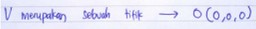Translated Version: V is a point that refers to O(0,0,0). (b)Student’s worksheet in PjBL class with no dynamic tool.

Figure 5. Sample students’ worksheets

The GeoGebra software application in the analytical geometry course gives significantly different visual illustrations compared with 2D sketches because GeoGebra can present dynamic graphic pictures (Caglayan, 2014; Haciomeroglu, 2011; Sangwin, 2007; Zekeriya & Douglas, 2011). This means that students can perceive the objects from various points of view. For example, prospective mathematics teachers more easily identify the particular position of a plane. This benefit is not obtained from a 2D visual illustration (additional steps are necessary to locate its position). The software helps teachers in problem solving and provides a variety of perspectives in solving problems (Nielsen & Solov’yov, 2019).

The Geo-PjBL model in this study emphasizes projects created by problem solvers to gain a better understanding of them. The project focuses on creating various visual illustration media with algebra software. The program can develop problems (Pradana et al., 2020) and alternative solutions for problem solving (Sangwin, 2007). Moreover, the prospective mathematics teacher can conceive analytical geometry content independently with no assistance from the lecturer. The Geo-PJBL model’s product is a collection of digital illustrations that can be reopened at any time for learning.

4. Conclusions

In general, the Geo-PjBL model has the potential to help prospective mathematics teachers gain an understanding of analytical geometry. Unfortunately, the results are not applicable to all subjects. The Geo-PJBL model is more effectively applied in analytical geometry subjects that require precision and accurate visual illustrations. Meanwhile, in the range of algebraic operations, the Geo-PjBL model is as effective as the PjBL model. Further research must develop more effective learning models when applied to subjects with the predominance of algebraic functions. This study used technology in the Geo-PJBL model, and the use of technology in the learning process is crucial. Lecturers are recommended to use software to provide visual illustrations of abstract mathematical objects.

5. Suggestions and Limitations

The PjBL model requires students to take an active role in creating learning media that fits their perspective. Moreover, GeoGebra is a supportive learning medium for the project. It is highly recommended that geometry teachers or lecturers use a virtual media–integrated learning model such as the Geo-PjBL model. The effort to employ students in a project that utilizes technology can hone their ICT skills as early as possible as a generation of Society 5.0. The students have to possess mathematics and ICT skills in making learning media with GeoGebra. In making shapes or media, they need to understand the mathematical equations inputted in the software. Otherwise, the resulting media will not be precise. For this reason, educators need to guide them in mathematics and ICT skills so that they can obtain superior achievement in this activity.

Declaration of Conflicting Interests

The authors declared no potential conflicts of interest with respect to the research, authorship, and/or publication of this article.

Funding

We are very grateful to Universitas Muhammadiyah Surakarta for financing our research through Grant Integration Tridharma (Hibah Integrasi Tridharma/HIT) in collaboration with Universitas PGRI Madiun in Indonesia.

References

Caglayan, G. (2014). Static Versus Dynamic Disposition: The Role of GeoGebra in Representing Polynomial-Rational Inequalities and Exponential-Logarithmic Functions. Computers in the Schools, 31(4), 339-370. https://doi.org/10.1080/07380569.2014.967632

Casa, T.M., Firmender, J.M., Gavin, M.K., & Carroll, S.R. (2016). Kindergarteners’ Achievement on Geometry and Measurement Units That Incorporate a Gifted Education Approach. Gifted Child Quarterly, 61(1), 52-72. https://doi.org/10.1177/0016986216671806

Crompton, H. (2015). Using Context-Aware Ubiquitous Learning to Support Students’ Understanding of Geometry. Journal of Interactive Media in Education, 1(13), 1–11. https://doi.org/10.5334/jime.aq

Fatahillah, A., Puspitasari, I.D., & Hussen, S. (2020). The Development of Schoology Web-Based Learning Media with Geogebra to Improve the ICT Literacy on Quadratic Functions. JRAMathEdu (Journal of Research and Advances in Mathematics Education), 5(3), 304-316. https://doi.org/10.23917/jramathedu.v5i3.10692

Fonna, M., & Mursalin, M. (2019). Using of Wingeom Software in Geometry Learning to Improving the of Mathematical Representation Ability. Malikussaleh Journal of Mathematics Learning (MJML), 1(2), 40-43. https://doi.org/10.29103/mjml.v1i2.1174

Haciomeroglu, E.S. (2011). Visualization Through Dynamic GeoGebra Illustrations. In Model-Centered Learning (133-144). Sense.

Hidayah, R., & Destari, T.Y. (2019). The Practicality of Scrap-mod as a Learning Media on Molecular Geometry. Proceedings of the Mathematics, Informatics, Science, and Education International Conference (MISEIC 2019), 95, 222-226. https://doi.org/10.2991/miseic-19.2019.52

Indahwati, R., & Tafrilyanto, C.F. (2018). Blog-Gothic As an Interactive Learning Media in Analytical Geometry Course. AKSIOMA: Jurnal Program Studi Pendidikan Matematika, 7(2), 266. https://doi.org/10.24127/ajpm.v7i2.1504

Janičić, P. (2006). GCLC A Tool for Constructive Euclidean Geometry and More Than That. International Congress on Mathematical Software, 4151, 58-73.

Kholid, M.N., Imawati, A., Swastika, A., Maharani, S., & Pradana, L.N. (2021). How are Students Conceptual Understanding for Solving Mathematical Problem?. In Journal of Physics: Conference Series (1776(1), 012018). IOP Publishing. https://doi.org/10.1088/1742-6596/1776/1/012018

Kholid, M.N., Sa’dijah, C., Hidayanto, E., & Permadi, H. (2020). How are Students’ Reflective Thinking for Problem Solving? Journal for the Education of Gifted Young Scientists, 8(3), 1135-1146. https://doi.org/10.17478/JEGYS.688210

Lin, H.C.K., Chen, M.C., & Chang, C.K. (2015). Assessing the effectiveness of learning solid geometry by using an augmented reality-assisted learning system. Interactive Learning Environments, 23(6), 799-810. https://doi.org/10.1080/10494820.2013.817435

Misrom, N.S., Abdurrahman, M.S., Abdullah, A.H., Osman, S., Hamzah, M.H., & Fauzan, A. (2020). Enhancing Students ’ Higher Order Thinking Skills (HOTS) Through an Inductive Reasoning Strategy Using Geogebra. International Journal of Emerging Technologies in Learning, 13(3), 156-179. https://doi.org/10.3991/ijet.v15i03.9839

Nielsen, C., & Solov’yov, I.A. (2019). MolSpinFlexible and Extensible General Spin Dynamics Software. AIP Conference Proceedings, 194105.

Pradana, L.N., Sholikhah, O.H., Maharani, S., & Kholid, M.N. (2020). Virtual Mathematics Kits (VMK): Connecting Digital Media to Mathematical Literacy. International Journal of Emerging Technologies in Learning (IJET), 15(3), 234-241. https://doi.org/10.3991/ijet.v15i03.11674

Sangwin, C. (2007). A Brief Review of GeoGebra: Dynamic Mathematics. MSOR Connections, 7(2), 36-38. https://doi.org/10.11120/msor.2007.07020036

Sinha, A., Bai, J., & Ramani, K. (2016). Deep Learning 3D Shape Surfaces Using Geometry Images. Lecture Notes in Computer Science (Including Subseries Lecture Notes in Artificial Intelligence and Lecture Notes in Bioinformatics), 9914 LNCS(VI), V. https://doi.org/10.1007/978-3-319-46466-4

Sholihah, U., & Maryono, M. (2020). Students Visual Thinking Ability in Solving the Integral Problem. Jramathedu (Journal of Research and Advances in Mathematics Education), 5(2), 175-186. https://doi.org/10.23917/jramathedu.v5i2.10286

Ummah, S.K., Inam, A., & Azmi, R.D. (2019). Creating Manipulatives: Improving Students’ Creativity Through Project-based Learning. Journal on Mathematics Education, 10(1), 93-102. https://doi.org/10.22342/jme.10.1.5093.93-102

Voronina, M.V., Moroz, O.N., Sudarikov, A.E., Rakhimzhanova, M.B., & Muratbakeev, E.K. (2017). Systematic Review and Results of The Experiment of A Flipped Learning Model for The Courses of Descriptive Geometry, Engineering and Computer Graphics, Computer Geometry. Eurasia Journal of Mathematics, Science and Technology Education, 13(8), 4831-4845. https://doi.org/10.12973/eurasia.2017.00967a

Zekeriya, K., & Douglas, M. (2011). Geogebra as a Cognitive Tool. In Model-Centered Learning (169-181). Sense.

Zhou, X., Wan, Q., Wei, Z., Xue, X., & Wei, Y. (2016). Model-based Deep Hand Pose Estimation. IJCAI International Joint Conference on Artificial Intelligence (2421-2427).